# Modal logic

(diff) ← Older revision | Latest revision (diff) | Newer revision → (diff)

The domain of logic in which along with the usual statements modal statements are considered, that is, statements of the type "it is necessary that …" , "it is possible that …" , etc. In mathematical logic various formal systems of modal logic have been considered, interrelations between these systems have been revealed, and their interpretations have been studied.

Elements of modal logic were in essence already known to Aristotle (4th century B.C.) and became part of classical philosophy. Modal logic was formalized for the first time by C.I. Lewis , who constructed five propositional systems of modal logic, given in the literature the notations S1–S5 (their formulations are given below). Other systems of modal logic were then constructed and investigated. The great variety of systems of modal logic is explained by the fact that the ideas of "possible" and "necessary" can be made precise in various ways; in addition, there are various ways to treat complex modalities (cf. Modality) of the type "necessarily possible" , and "interrelations" of modality with the logical connectives. The majority of systems of modal logic which have been studied are based on classical logic; however, systems based on intuitionistic logic have also been discussed (see, for example, ).

Below, several of the most widely-studied propositional systems of modal logic are described. The language of each of these systems is obtained from the language of classical propositional calculusby the addition of the new one-place connectives (modal operators)(necessary) and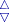(possible). Since in almost all these systems the relation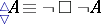(*)

holds, initially one modal operator is chosen, for example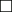, and the other is defined by (*).

The system S1. Axiom schemes:

1) all formulas of the form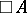, whereis a formula derivable in;

2)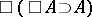;

3)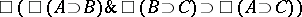.

Derivation rules:

I)(modus ponens);

II)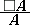;

III)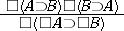.

The system S2: S1 +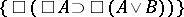.

The system S3: S2 +.

The system K. Axiom schemes:

1) the axiom schemes of the propositional calculus;

2).

Derivation rules: modus ponens and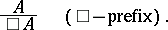The system T: K +S2 +.

The system B: T +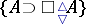.

The system S4: S3 +T +.

The system S5: S4 +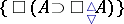.

Among the above systems, S4 has important significance since the intuitionistic propositional calculuscan be interpreted in it, that is, with respect to every propositional (non-modal) formulait is possible to construct a formulaof modal logic such that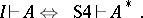In this connection the system of Grzegorczyk is of particular interest (see ):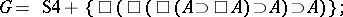for it the transference theorem is true: For any set of axiom schemesand any formula,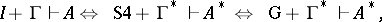where; moreover, G is the strongest system with this property. This theorem makes it possible to transfer a property (for example, completeness or decidability) from an extension of the system S4 (or G) to an intermediate logic.

For each propositional system of modal logic S it is possible to consider the corresponding predicate system, which is obtained by the addition of object variables, predicate symbols and the quantifiers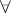,(or one of these) to the language of S. The usual axiom schemes and derivation rules for quantifiers are added. In addition one sometimes also adds axioms which describe the actions of modal operators on quantifiers such as, for example, the Barcan formula:The algebraic interpretation of a system of modal logic is given by some algebra (also called a matrix)whereis the set of truth values (cf. Truth value),is a set of distinguished truth values,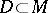, and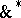,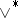,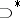,,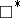are the operations incorresponding to the connectives,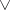,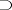,,. A formula is called generally valid inif for every valuation of its propositional variables by elements ofit takes a distinguished value. A system of modal logic S is called complete relative to a class of algebrasif a formula is derivable in S if and only if it is generally valid in every algebra in the class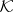. For example, the system S4 is complete relative to the class of so-called finite topological Boolean algebras (see ). In general, a system S is called finitely approximable if it is complete relative to finite algebras. If a system is finitely axiomatizable and finitely approximable, then it is decidable, that is, the problem of recognition of derivability is algorithmically decidable. A matrixis called characteristic or adequate for a system S if S is complete relative to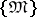. None of the above-mentioned propositional systems of modal logic has a finite adequate matrix, but each of them is finitely approximable and therefore decidable. On the other hand, every extension of S5 has a finite adequate matrix with one distinguished value. The property of finite approximability also holds for all extensions of the systemAn important tool in the study of modal logics are Kripke models, having the form, whereis a set (called the set of "worlds" , "situations" ),is a relation onandis a valuation of the propositional variables by subsets of. For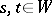the relationcan be treated as "the world t is possible in the world s" . The pair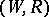is called a Kripke structure, or frame (the term scale is also used). A formulais generally valid in the frameif for each valuationformulais true in the Kripke model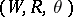. A system S is called Kripke complete relative to a class of Kripke structures if the S-derivable formulas are exactly the formulas which are generally valid in all Kripke structures in the class. For example, the system T is Kripke complete relative to the class of structures, whereis a reflexive relation; S4 is Kripke complete relative to a structure with a reflexive and transitive relation. Among the finitely-axiomatizable extensions of S4 there are extensions which are not Kripke complete (see ).

For predicate systems of modal logic the Kripke models have the form, where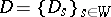,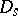is a universe for the world,is an interpretation of the predicate symbols in, andis a valuation associating to object variables some elements of the set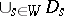. For systems containing the Barcan formula, it is also necessary to require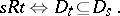Kripke models, as a rule, have a more easily visualized structure than algebraic models; therefore they are often more convenient for the study of different systems of modal logic.

How to Cite This Entry:
Modal logic. Encyclopedia of Mathematics. URL: http://encyclopediaofmath.org/index.php?title=Modal_logic&oldid=12997
This article was adapted from an original article by S.K. Sobolev (originator), which appeared in Encyclopedia of Mathematics - ISBN 1402006098. See original article